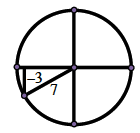### Home > PC3 > Chapter 10 > Lesson 10.1.4 > Problem10-87

10-87.

If $\sin(x) = −\frac { 3 } { 7 }$, $π ≤ x ≤ \frac { 3 \pi } { 2 }$, calculate exact values for $\sin(2x)$ and $\cos(2x)$.

Draw and label a circle with the reference angle in the correct quadrant.
Calculate the length of the unlabeled side.

Double - Angle Formula: Sine
$\sin(2α) = 2\sin(α)\cos(α)$

Double - Angle Formulas: Cosine
1. $\cos(2α) = 1 − 2\sin^2(α)$
2. $\cos2(α) = 2\cos2(α) − 1$
3. $\cos(2α) = \cos^2(α) − \sin^2(α)$